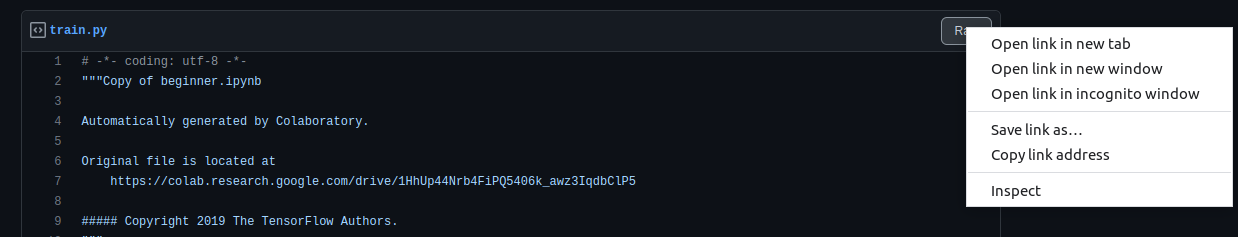# Training Tensorflow ModelTensorFlow is an open-source machine learning software library, TensorFlow is used to train neural networks. Expressed in the form of stateful dataflow graphs, each node in the graph represents the operations performed by neural networks on multi-dimensional arrays. These multi-dimensional arrays are commonly known as “tensors,” hence the name TensorFlow. In this example, we will be training a MNIST model.

## TD;lR​

Running any type of Tensorflow model with Bacalhau

## Training TensorFlow models Locally​

This section is from TensorFlow 2 quickstart for beginners

### TensorFlow 2 quickstart for beginners​

This short introduction uses Keras to:

2. Build a neural network machine learning model that classifies images.
3. Train this neural network.
4. Evaluate the accuracy of the model.

### Set up TensorFlow​

Import TensorFlow into your program to check whether it is installed

``import tensorflow as tfimport osprint("TensorFlow version:", tf.__version__)``
``%%bashmkdir /inputswget https://storage.googleapis.com/tensorflow/tf-keras-datasets/mnist.npz -O /inputs/mnist.npz``
``mnist = tf.keras.datasets.mnistCWD = '' if os.getcwd() == '/' else os.getcwd()(x_train, y_train), (x_test, y_test) = mnist.load_data('/inputs/mnist.npz')x_train, x_test = x_train / 255.0, x_test / 255.0``

### Build a machine-learning model​

Build a `tf.keras.Sequential` model by stacking layers.

``model = tf.keras.models.Sequential([  tf.keras.layers.Flatten(input_shape=(28, 28)),  tf.keras.layers.Dense(128, activation='relu'),  tf.keras.layers.Dropout(0.2),  tf.keras.layers.Dense(10)])``

For each example, the model returns a vector of logits or log-odds scores, one for each class.

``predictions = model(x_train[:1]).numpy()predictions``

The `tf.nn.softmax` function converts these logits to probabilities for each class:

``tf.nn.softmax(predictions).numpy()``

Note: It is possible to bake the `tf.nn.softmax` function into the activation function for the last layer of the network. While this can make the model output more directly interpretable, this approach is discouraged as it's impossible to provide an exact and numerically stable loss calculation for all models when using a softmax output.

Define a loss function for training using `losses.SparseCategoricalCrossentropy`, which takes a vector of logits and a `True` index and returns a scalar loss for each example.

``loss_fn = tf.keras.losses.SparseCategoricalCrossentropy(from_logits=True)``

This loss is equal to the negative log probability of the true class: The loss is zero if the model is sure of the correct class.

This untrained model gives probabilities close to random (1/10 for each class), so the initial loss should be close to `-tf.math.log(1/10) ~= 2.3`.

``loss_fn(y_train[:1], predictions).numpy()``

Before you start training, configure and compile the model using Keras `Model.compile`. Set the `optimizer` class to `adam`, set the `loss` to the `loss_fn` function you defined earlier, and specify a metric to be evaluated for the model by setting the `metrics` parameter to `accuracy`.

``model.compile(optimizer='adam',              loss=loss_fn,              metrics=['accuracy'])``

### Train and evaluate your model​

Use the `Model.fit` method to adjust your model parameters and minimize the loss:

``model.fit(x_train, y_train, epochs=5)``

The `Model.evaluate` method checks the models performance, usually on a "Validation-set" or "Test-set".

``model.evaluate(x_test,  y_test, verbose=2)``

The image classifier is now trained to ~98% accuracy on this dataset. To learn more, read the TensorFlow tutorials.

If you want your model to return a probability, you can wrap the trained model, and attach the softmax to it:

``probability_model = tf.keras.Sequential([  model,  tf.keras.layers.Softmax()])``
``probability_model(x_test[:5])``
``%%bashmkdir /outputs``

the following method can be used to save the model as a checkpoint

``model.save_weights('/outputs/checkpoints/my_checkpoint')``
``%%bashls /outputs/``

### Converting the notebook into a Python script​

You can use a tool like `nbconvert` to convert your Python notebook into a script.

After that, you can create a gist of the training script at gist.github.com copy the raw link of the gist``%%bashwget https://gist.githubusercontent.com/js-ts/0ce4d671ced642fbe807e65f5186ae87/raw/7f28cc497cc1c509661a33b144c0683b8fc97f41/train.py``

Testing whether the script works

``%%bashpython train.py``

## Running on bacalhau​

``!curl -sL https://get.bacalhau.org/install.sh | bash``

The dataset and the script are mounted to the TensorFlow container using an URL we then run the script inside the container

``%%bash --out job_idbacalhau docker run \--wait \--id-only \-w /inputs  \-i https://gist.githubusercontent.com/js-ts/e7d32c7d19ffde7811c683d4fcb1a219/raw/ff44ac5b157d231f464f4d43ce0e05bccb4c1d7b/train.py \-i https://storage.googleapis.com/tensorflow/tf-keras-datasets/mnist.npz \tensorflow/tensorflow \-- python train.py``

Structure of the command:

• `-i https://gist.githubusercontent.com/js-ts/e7d32c7d19ffde7811c683d4fcb1a219/raw/ff44ac5b157d231f464f4d43ce0e05bccb4c1d7b/train.py`: mount the training script

• `-i https://storage.googleapis.com/tensorflow/tf-keras-datasets/mnist.npz`: mount the dataset

• `tensorflow/tensorflow`: specify the Docker image

• `python train.py`: execute the script

By default whatever URL you mount using the -i flag gets mounted at the path /inputs so we choose that as our input directory `-w /inputs`

``%%bashbacalhau list --id-filter \${JOB_ID}``

Where it says `Completed`, that means the job is done, and we can get the results.

``%%bashbacalhau describe \${JOB_ID}``
``%%bashrm -rf results && mkdir -p resultsbacalhau get \$JOB_ID --output-dir results``
``%%bashls results/``
``%%bashcat results/stdout``
``%%bashls results/outputs/``